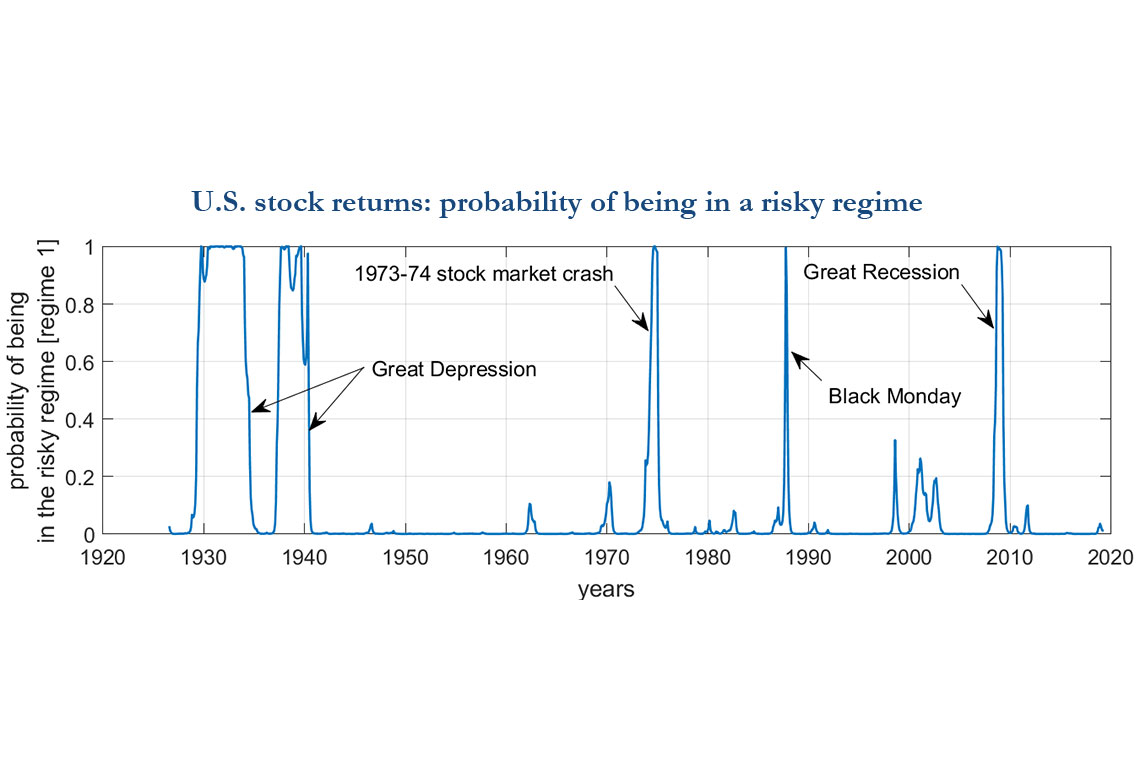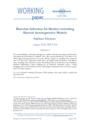# Working Paper Series no. 726: Bayesian Inference for Markov-switching Skewed Autoregressive Models

We examine Markov-switching autoregressive models where the commonly used Gaussian assumption for disturbances is replaced with a skew-normal distribution. This allows us to detect regime changes not only in the mean and the variance of a specified time series, but also in its skewness. A Bayesian framework is developed based on Markov chain Monte Carlo sampling. Our informative prior distributions lead to closed-form full conditional posterior distributions, whose sampling can be efficiently conducted within a Gibbs sampling scheme. The usefulness of the methodology is illustrated with a real-data example from U.S. stock markets.Markov-switching models have been a popular tool used in econometric time series analysis. So far almost all extensions and applications of these models have been devoted to detecting abrupt changes in the behavior of the first two moments of a given series --- namely, the mean and the variance. Notable examples include Hamilton (1989)’s model of long-term mean rate of economic growth; stock return volatility models of Turner, Startz, and Nelson (1989) and Pagan and Schwert (1990), the exchange rate dynamics model of Engel and Hamilton (1990) and the real interest rate model of Garcia and Perron (1996).
By contrast, skewness, the third moment, has received little attention in the Markov-switching literature, though it is found in many economic time series, such as stock returns and exchange rate returns, and it appears to vary over time (see, for example, Alles and Kling (1994) and Harvey and Siddique (1999) for analysis of U.S. monthly stock indices, and Johnson (2002), Carr and Wu (2007), and Bakshi, Carr, and Wu (2008) for analysis of exchange rate returns). This important omission is due to the commonly used Gaussian assumption for disturbances, which does not allow for possible departure from symmetry.
In this paper, we work with the autoregressive time series (AR) model with Markov-switching introduced by Hamilton (1989), but relax the normality assumption. Instead, we consider a skew-normal distribution proposed by Azzalini (1985, 1986). The key innovation in his work is to account for several degree of asymmetry. With respect to the normal distribution, the skew-normal family is a class of density functions that depends on an additional shape parameter that affects the tails of the density. Such a distribution has already been intensively studied in statistics, biologists, engineers, and medical researchers, but remains largely unexplored in economics.
Our approach here is to propose a simple and easy-to-implement Bayesian framework for such models. More specifically, we develop a Gibbs sampler for Bayesian inferences of AR time series subject to Markov mean, variance and skewness shifts. Our Gibbs sampling procedure can be seen as an extension of Albert and Chib (1993) to account for time variations in the skewness. Specifically, we take advantage of the stochastic representation of skew-normal variables, which is based on a convolution of normal and truncated-normal variables, in order to obtain a straightforward Markov Chain Monte Carlo (MCMC) sampling sequence that involves a 7-block Gibbs sampler for Markov-switching models, in which one can generate in a flexible and straightforward manner alternatively draws from full conditional posterior distributions. In order to make computationally feasible estimation and inference, we provide a companion software package for anyone interested in such models.
As an empirical illustration of our approach, we analyze the time-varying distribution of NYSE/AMEX/NASDAQ stock index returns. We establish two regimes. The first, prevailing during the periods of financial distress, is marked by negative expected returns, large conditional volatility, and positive conditional skewness. The second, frequently observed during tranquil periods, exhibits positive expected returns, low conditional volatility, and negative conditional skewness. Therefore, our result shows that stocks are particularly risky to hold in bad times, like the Great Recession. However, during such times, the positive degree of skewness, indicating that extreme values on the right side of the mean are more likely than the extreme values of the same magnitude on the left side of the mean, allow sometimes to perform large positive returns. Say it differently, stocks produce negative average returns in bad times, but sometimes take large positive hits